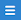## Saturday, 20 May 2023

### Using the Calc Object in nuBuilder Forte

#### The Calc Object

The Calc Object in nuBuilder makes a nuBuilder Form work a little like a spreadsheet by creating Objects that are automatically updated anytime certain other Objects are edited or updated.

In some cases the Calc Object is updated from other Calc Objects.

In cases like this, you will need to make sure they are being calculated in the correct order.

Otherwise, you can get into a situation like this...

There are 4 Calc Objects on this invoice Form and this is what they calculate...

Unit TotalnuTotal('iit_units') * nuTotal('iit_unit_price')             eg. Units * Unit Price

Total: nuTotal('item_sf.iit_unit_total')                                         eg. Sum of all Unit Total

Tax: nuTotal('inv_total') *  ( nuTotal('inv_tax_percent') * .01 )    eg. Total * Tax %

Grand Total: nuTotal('inv_total') + nuTotal('inv_tax_total')        eg. Total + Tax

In the example above Grand Total is calculated before Total Units, Total and Tax, which isn't correct.

To make Grand Total calculate correctly it must be calculated last and to reorder this we need to select Arrange Objects from the Form's menu () and move Grand Total down below Tax Total.

Then all the Calc Objects will calculate properly...

Reordering any Objects will also reorder the tabbing order of those Objects on the Form.